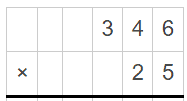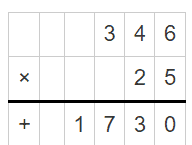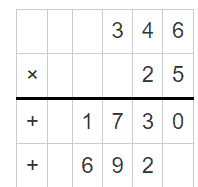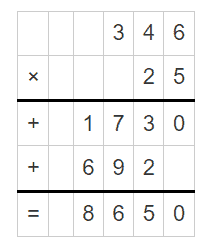# 2 digit by 3 digit Multiplication Worksheets

• ###### 2 digit by 3 digit Multiplication #15

Two-digit by three-digit multiplication worksheets are valuable to help primary level students grasp the concept of multi-digit multiplication. They serve as an important step in building a student’s mathematical foundation, transitioning from simple multiplication problems (like multiplying single digits) to more complex calculations involving larger numbers.

These worksheets contain exercises where a two-digit number (like 25) is multiplied by a three-digit number (like 123). It’s a more intricate process compared to multiplying single-digit numbers, and these worksheets aim to help students understand and master the procedure.

Two-digit by three-digit multiplication worksheets follow a specific structure that promotes a step-by-step understanding. The multiplication problem is often set up in the traditional format, with the three-digit number on top, the two-digit number beneath it, and a line drawn under both numbers. The student is then tasked with multiplying each digit of the two-digit number with every digit of the three-digit number, carrying over any values that exceed ten to the next column. Finally, the student adds up the resulting numbers to find the final product.

Here’s how these worksheets can significantly improve a student’s math skills:

Mastery of Basic Operations – At their core, these worksheets help solidify the understanding of basic multiplication. While the numbers are larger, the basic operation is still multiplication, which is an essential mathematical skill.

Arithmetic Skills – Two-digit by three-digit multiplication involves a blend of multiplication and addition. The process of carrying over numbers and adding the results refines the student’s arithmetic skills.

Problem-solving Skills – Each multiplication problem can be seen as a mini puzzle. It requires breaking down a complex problem into simpler steps, a key problem-solving strategy that’s not just applicable to mathematics but to a broad range of challenges students may face in their academic journey.

Cognitive Development – Working through these worksheets can help enhance cognitive abilities such as attention to detail, memory, logical reasoning, and critical thinking skills. It involves remembering multiplication tables, carefully executing each step, and checking the result for errors.

Confidence with Large Numbers – Multiplication worksheets with larger numbers can help reduce any intimidation students might feel when dealing with larger numbers, making them more comfortable and confident in handling more complex calculations in the future.

Preparation for Advanced Mathematics – Mastery of multi-digit multiplication is critical for advanced mathematical concepts such as algebra, calculus, and beyond. These worksheets lay a strong foundation for future math studies.

Real-world Applications – Many real-world situations require the ability to multiply large numbers, such as calculating prices, determining areas, understanding statistics, or estimating quantities. Therefore, practicing with these worksheets can enhance students’ abilities to apply math in real-world scenarios.

Persistence – These worksheets also teach persistence. It takes time and patience to work through multi-step multiplication problems. Learning to stick with a problem until it’s solved is a valuable life skill.

### How Do You Multiply 2 Digit Numbers by 3 Digit Numbers?

We will explain through the example of multiplying the two-digit number 25 by the three-digit number 346.

Step 1: Write down the numbers vertically, lining up the digits according to their place value.Step 2: Start by multiplying the ones digit of the two-digit number (5) by each digit of the three-digit number, working from right to left.Step 3: Multiply the tens digit of the two-digit number (2) by each digit of the three-digit number, again working from right to left.Step 4: Add up all the partial products to get the final result.